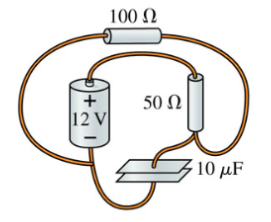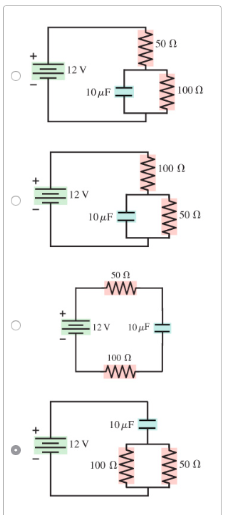# Problem: Draw the circuit diagram for the circuit of (Figure 1).Choose the correct options.

###### FREE Expert Solution

From the positive terminal, we meet the 50Ω resistor. After the resistor, the circuit branches into 2:

87% (457 ratings)###### Problem Details

Draw the circuit diagram for the circuit of (Figure 1).Choose the correct options.Frequently Asked Questions

What scientific concept do you need to know in order to solve this problem?

Our tutors have indicated that to solve this problem you will need to apply the !! Resistor-Capacitor Circuits concept. If you need more !! Resistor-Capacitor Circuits practice, you can also practice !! Resistor-Capacitor Circuits practice problems.